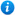* Watermarks do not appear on purchased resources

Preview resource: Click slides to enlarge

# Division with Remainders - Year 3

Division with Remainders - Year 3

Year 3 maths programme of study - Number - Number (multipliction and division)

• recall and use multiplication and division facts for the 3, 4 and 8 multiplication tables
• write and calculate mathematical statements for multiplication and division using the multiplication tables that they know
• solve problems, including missing number problems, involving multiplication and division
In this maths teaching resource, pupils practise dividing 2-digit numbers by a 1-digit number with a remainder. These activities cover the year 3 curriculum objectives listed above and support the White Rose small steps guidance for year 3 - Spring - Block 1 - Multiplication and Division. The resource consists of 1 lesson, including a PowerPoint presentation and differentiated worksheets. The worksheets contain varied fluency, reasoning and problem solving to reinforce and consolidate the children's learning. Content includes:

• Varied fluency and reasoning and problem solving interactive class activities
• 3 differentiated varied fluency and reasoning and problem solving worksheets with answers

'Division with Remainders - Year 3' is completely editable giving teachers the freedom to adapt the resource to suit their individual teaching needs.

Click on the images from the PowerPoint presentation to view the resource in more detail.

Our Price : £2.99 / 3 Credits